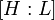Generalized dihedral group

Definition

Suppose$H$ is an abelian group. The generalized dihedral group corresponding to$H$ is the external semidirect product of$H$ with the cyclic group of order two, with the non-identity element acting as the inverse map on$H$.

Viewing this external semidirect product as an internal semidirect product,$H$ is an abelian normal subgroup of index two.

A presentation for$G$ is:$G := \langle H,x \mid xhx^{-1} = h^{-1} \forall h \in H \rangle$.

Note that the dihedral groups are special cases of generalized dihedral groups where the abelian group in question is a cyclic group.

Arithmetic functions

Function Value Explanation
order Twice the order of$H$
exponent Least common multiple of$2$ and the exponent of$H$
derived length$1$ if$H$ is an elementary abelian$2$-group,$2$ otherwise.
nilpotency class Frattini length of$H$ if$H$ is a$2$-group, not defined otherwise.
max-length One more than the max-length of$H$.
composition length One more than the composition length of$H$.
chief length One more than the chief length of$H$.
minimum size of generating set One more than the minimum size of generating set of$H$.
number of subgroups number of subgroups of$H$ plus sum of indices of subgroups of$H$.
number of conjugacy classes$(n + 3 \cdot 2^k)/2$ where$n = |H|$ and$2^k = |H/S|$ where$S$ is the set of squares in$H$.

Group properties

Property Satisfied Explanation
Abelian group True only if$H$ is an elementary abelian$2$-group
Nilpotent group True only if$H$ is a$2$-group
Solvable group Yes
Metabelian group Yes

Subgroups

Further information: Subgroup structure of generalized dihedral groups

There are two kinds of subgroups of the generalized dihedral group$G$ with the abelian subgroup$H$:

1. Subgroups of$H$: All of these are normal subgroups of$G$. The number of such subgroups equals the number of subgroups of$H$.
2. Subgroups of$G$ containing an element outside$H$: Suppose$K$ is such a subgroup. Then$L =H \cap K$ is a subgroup of index two in$K$, and$K$ is the union of$L$ and a coset$gL$ where$g \in G \setminus H$, i.e.,$g \in G, g \notin H$. Thus, to specify$K$, it suffices to specify$L$ and the coset$gL$. Conversely, given any subgroup$L \le H$ and any coset$gL$ with$g \in G \setminus H$, we obtain a subgroup$K = L \cup gL$. The reason this is a subgroup is because$g$ has order two and acts on$L$ by the inverse map. The upshot is that, given$L$, the number of possibilities for$K$ is the number of cosets of$L$ outside$H$, which equals the number of cosets inside$H$, which equals the index$[H:L]$. Thus, the total number of possibilities for$K$ is the sum of subgroup indices over all subgroups of$H$.